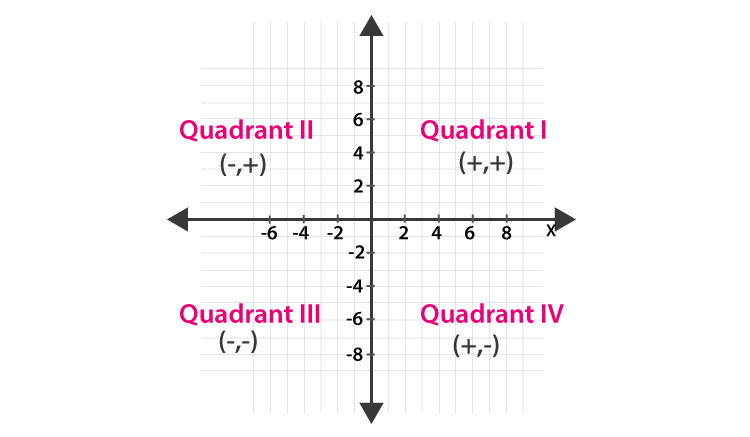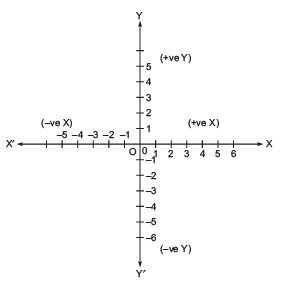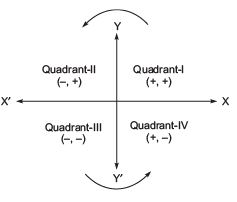Courses

Short Notes - Coordinate Geometry Notes | Study Class 9 Mathematics by VP Classes - Class 9

Class 9: Short Notes - Coordinate Geometry Notes | Study Class 9 Mathematics by VP Classes - Class 9

The document Short Notes - Coordinate Geometry Notes | Study Class 9 Mathematics by VP Classes - Class 9 is a part of the Class 9 Course Class 9 Mathematics by VP Classes.
All you need of Class 9 at this link: Class 9

Facts that MatterCoordinate plane

• The position of a point is located on a plane by drawing two lines mutually perpendicular to each other.
• The plane is called the cartesian or coordinate plane and the mutually perpendicular lines are called axes.
• The horizontal line is called the x-axis and the vertical line is called the y-axis.
• The 'x-coordinate' of a point is called the abscissa.
• The 'y-coordinate' of a point is called the ordinate.
• The abscissa of every point is 0 on the y-axis and the ordinate of every point is 0 on the x-axis.
• The coordinates of the origin are (0, 0).
• The axes divide the plane into four quadrants.
• The points of the type: (+, +) lies in the quadrant I (–, +) lies in the quadrant II (–, –) lies in the quadrant III (+, –) lies in the quadrant IV.
• We can represent a point in plane (called a cartesian plane or a coordinate plane) by means of an ordered pair of real numbers, called the coordinates of that point.
• The branch of mathematics in which geometric problems are solved using coordinate systems is known as Coordinate Geometry.

Try yourself:The axes divide the coordinate plane into four sections called what?

Note:
(i) An ordered pair means two numbers ‘x’ and ‘y’ listed in a specific order such that ‘x’ is placed at the first place and ‘y’ is placed at the second place. We write an ordered pair as (x, y).
(ii) (x, y) is one ordered pair and (y, x) is another ordered pair.
The position of a point in a plane is determined with reference to two fixed mutually perpendicular lines, called the coordinate axes. The horizontal line is called the x-axis and the vertical line is called the y-axis.

Cartesian System

• Two number lines mutually perpendicular to each other are called axes.
• The horizontal line XOX' and called the x-axis.
• The vertical line YOY', is called the y-axis.
• Both these lines are in the same plane, called the ‘cartesian plane’ or ‘coordinate plane’ or the ‘XY-plane’.Let us note the following points:
(i) The perpendicular distance of a point from the y-axis is called its x-coordinate (or abscissa).
(ii) The perpendicular distance of the point from the x-axis is called its y-coordinate (or its ordinate)
(iii) The abscissa of every point on y-axis is zero.
(iv) The ordinate of every point on the x-axis is zero.
(v) The axes intersect at a point called the origin (i.e. x-axis and y-axis intersect at the origin)
(vi) The coordinates of the origin are (0, 0).

Note:
The positive numbers lie on the directions OX and OY. So OX and OY are positive directions of x-axis and y-axis respectively. Similarly OX' and OY' are called the negative directions of the x-axis and the y-axis respectively.

The coordinate axes divide the plane into four parts called quadrants (one-fourth part), numbered I, II, III, and IV anticlockwise from OX.The coordinates are of the form:
(i) (+, +) in the I-quadrant.
(ii) (–, +) in the II-quadrant.
(iii) (–, –) in the III-quadrant.
(iv) (+, –) in the IV-quadrant.

Try yourself:If the coordinates of a point are (-3,4), then it lies in:

➢ Plotting a Point in the Plane when Coordinates of the Point are Given

To plot a point in the coordinate plane:
(i) We draw the coordinate axes and choose our units such that we can mark equal distances on the x-axis or on the y-axis or on both axes.
(ii) We take origin as zero on the x-axis as well as on the y-axis.
(iii) The distances marked along OX and OY are taken as positive and those along OX' and OY' are taken as negative.

The document Short Notes - Coordinate Geometry Notes | Study Class 9 Mathematics by VP Classes - Class 9 is a part of the Class 9 Course Class 9 Mathematics by VP Classes.
All you need of Class 9 at this link: Class 9Use Code STAYHOME200 and get INR 200 additional OFF Use Coupon Code

Top Courses for Class 9Class 9 Mathematics by VP Classes

125 docs

Top Courses for Class 9Track your progress, build streaks, highlight & save important lessons and more!

,

,

,

,

,

,

,

,

,

,

,

,

,

,

,

,

,

,

,

,

,

;